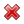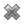# Fight Finance

#### CoursesTagsRandomAllRecentScoresScoreskeithphw $6,011.61 Jade$1,815.80 Chu $789.98 royal ne...$750.00 Leehy $713.33 Visitor$650.00 JennyLI $625.61 Visitor$590.00 Visitor $550.00 Visitor$550.00 ZOE HY $540.00 Visitor$540.00 Visitor $500.00 Yizhou$489.18 Visitor $464.70 Jasper.sun$460.00 Visitor $460.00 Visitor$460.00 Visitor $442.43 niki,zhang$440.00

One year ago a pharmaceutical firm floated by selling its 1 million shares for $100 each. Its book and market values of equity were both$100m. Its debt totalled $50m. The required return on the firm's assets was 15%, equity 20% and debt 5% pa. In the year since then, the firm: • Earned net income of$29m.
• Paid dividends totaling \$10m.
• Discovered a valuable new drug that will lead to a massive 1,000 times increase in the firm's net income in 10 years after the research is commercialised. News of the discovery was publicly announced. The firm's systematic risk remains unchanged.

Which of the following statements is NOT correct? All statements are about current figures, not figures one year ago.

Hint: Book return on assets (ROA) and book return on equity (ROE) are ratios that accountants like to use to measure a business's past performance.

$$\text{ROA}= \dfrac{\text{Net income}}{\text{Book value of assets}}$$

$$\text{ROE}= \dfrac{\text{Net income}}{\text{Book value of equity}}$$

The required return on assets $r_V$ is a return that financiers like to use to estimate a business's future required performance which compensates them for the firm's assets' risks. If the business were to achieve realised historical returns equal to its required returns, then investment into the business's assets would have been a zero-NPV decision, which is neither good nor bad but fair.

$$r_\text{V, 0 to 1}= \dfrac{\text{Cash flow from assets}_\text{1}}{\text{Market value of assets}_\text{0}} = \dfrac{CFFA_\text{1}}{V_\text{0}}$$

Similarly for equity and debt.